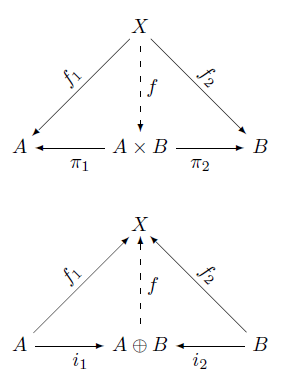# Commutative diagrams in LaTeX

There are numerous packages for creating commutative diagrams in LaTeX. My favorite, based on my limited experience, is Paul Taylor’s package. Another popular package is tikz-cd.

To install Paul Taylor’s package on Windows, I created a directory called localtexmf, set the environment variable TEXINPUTS to its location, and copied diagrams.sty file in that directory.

Here are a couple examples, diagrams used in the definition of product and coproduct.And here’s the LaTeX to produce the diagrams.

\begin{diagram}
& & X & & \\
& \ldTo^{f_1} & \dDashto_f & \rdTo^{f_2} & \\
A & \lTo_{\pi_1} & A\times B & \rTo_{\pi_2} & B \\
\end{diagram}

\begin{diagram}
& & X & & \\
& \ruTo^{f_1} & \uDashto_f & \luTo^{f_2} & \\
A & \rTo_{i_1} & A\oplus B & \lTo_{i_2} & B \\
\end{diagram}